Plotting Ontological Terms

2016-10-27

ontologyPlot is part of the ‘ontologyX’ family of packages (see the ‘Introduction to ontologyX’ vignette supplied with the ontologyIndex package). It enables visualisation of ontological terms and ontological annotation as subgraphs of the full ontology, rendered using the Rgraphviz package. The package makes use of ontology_index objects, provided by the ontologyIndex package. To make full use of the features in ontologyPlot, the user is encouraged to gain familiarity of the functions in ontologyIndex which enable various transformations of sets of ontological that are useful for selecting those to add to diagrams.

Note: Rgraphviz must be obtained from the Bioconductor repository, http://bioconductor.org/packages/Rgraphviz/.

We start by loading both packages, and an example ontology - the Human Phenotype Ontology (HPO).

library(ontologyIndex)
library(ontologyPlot)
data(hpo)

Plotting Terms

A set of terms (character vector of term IDs) can be plotted as a subgraph of the ontology by calling onto_plot:

onto_plot(hpo, terms=c("HP:0001873", "HP:0011877"))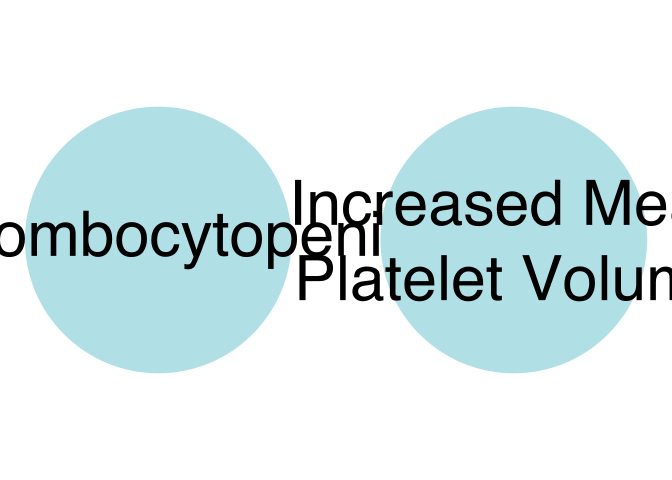To include all ancestral terms, use the get_ancestors function:

onto_plot(hpo, terms=get_ancestors(hpo, c("HP:0001873", "HP:0011877")))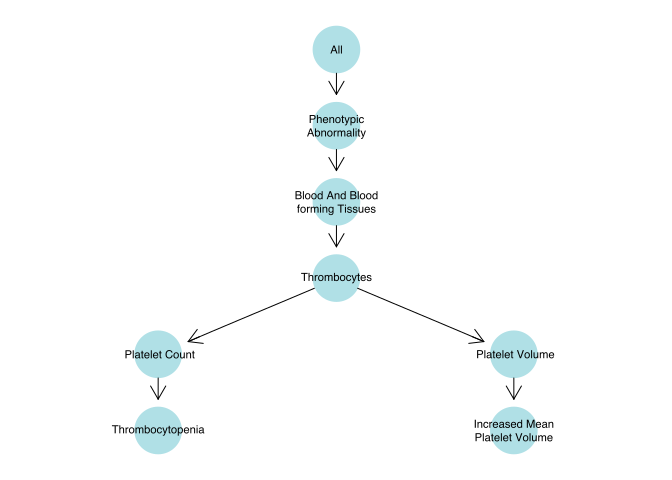The function remove_links can be used to remove terms simply linking two terms together, thus the structure of the given part of the ontology can be retained whilst simplifying the figure:

onto_plot(hpo, terms=remove_links(hpo, get_ancestors(hpo, c("HP:0001873", "HP:0011877"))))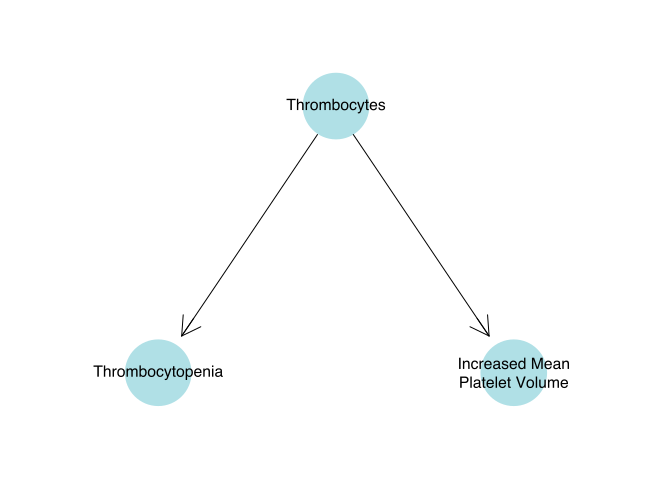Graphical parameters

Graphical attributes to assign to each node can be passed to onto_plot. The graphical attributes can be specified as:

• a single value, thus setting the attribute to the given value for all nodes,
• a vector the same length as the terms argument, so the ith term gets the ith attribute value,
• a function which returns the desired attribute value for each term.

Node attributes which affect the plot generated include those supported by the Rgraphviz package. Some of these include:

• fillcolor - the colour to use for each node,
• color - the colour for the border of the node (defaults to "transparent"),
• label - a character vector of term IDs to be used as the labels,
• fontsize - font size of label text printed in each term,
• width - the relative width of the nodes.

In this example, we pass the term IDs as the labels and a character vector of colours generated with rainbow to colour the nodes.

terms <- remove_links(hpo, get_ancestors(hpo, c("HP:0001873", "HP:0011877")))
onto_plot(hpo, terms=terms, label=terms, fillcolor=rainbow(length(terms)))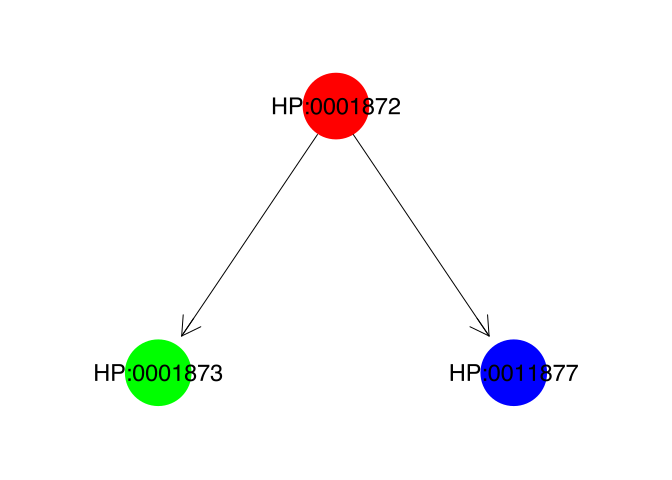ontologyPlot contains several functions for setting the graphical parameters which can be passed to onto_plot. These include for example label_by_frequency, official_labels and label_by_term_set (see individual help files for more details).

These functions must accept an ontology and terms argument. They can optionally accept:

• frequencies: a numeric vector of population frequencies named by term,
• term_sets: a list of character vectors of terms [i.e. annotated objects] arguments.

If any of the given functions require these additional arguments, they must be passed to onto_plot.

Frequencies

A frequencies argument giving a population frequency of terms can be passed to onto_plot. If this argument is passed, functions which make use of it in determining graphical parameters can be passed to onto_plot, for instance colour_by_population_frequency.

frequencies <- seq(from=0, to=1, by=1/length(terms))
names(frequencies) <- terms
onto_plot(hpo, terms=terms, frequencies=frequencies,
fillcolor=colour_by_population_frequency)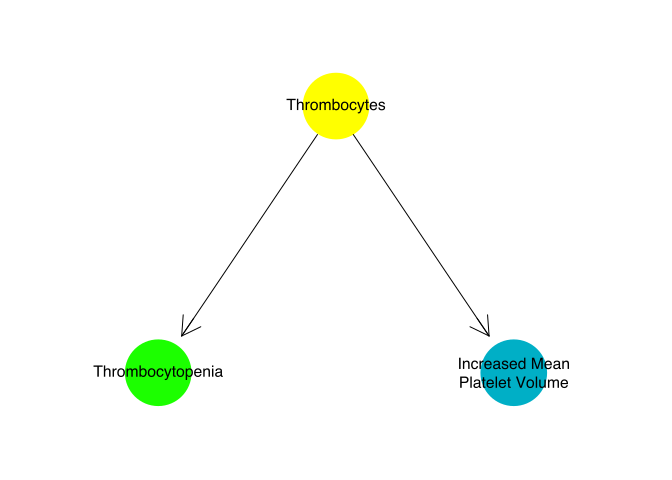Term sets

Another argument which can be passed to onto_plot is term_sets, a list of sets of ontological terms (e.g. a set of HPO encoded phenotypes). If terms_sets is given, the terms parameter of onto_plot defaults to the value of remove_uninformative_terms(ontology, term_sets), which removes terms which are annotated to exactly the same objects as all of their children (with the effect of greatly simplifying the resulting diagram - see ?remove_uninformative_terms for more details).

hpo_phenotypes <- list(
A=c("HP:0001382","HP:0004272","HP:0007917","HP:0004912","HP:0001596"),
B=c("HP:0001382","HP:0004272","HP:0002165","HP:0004800","HP:0004912"),
C=c("HP:0004800","HP:0001382","HP:0004912","HP:0007917","HP:0008743")
)

onto_plot(hpo, term_sets=hpo_phenotypes, label=label_by_term_set)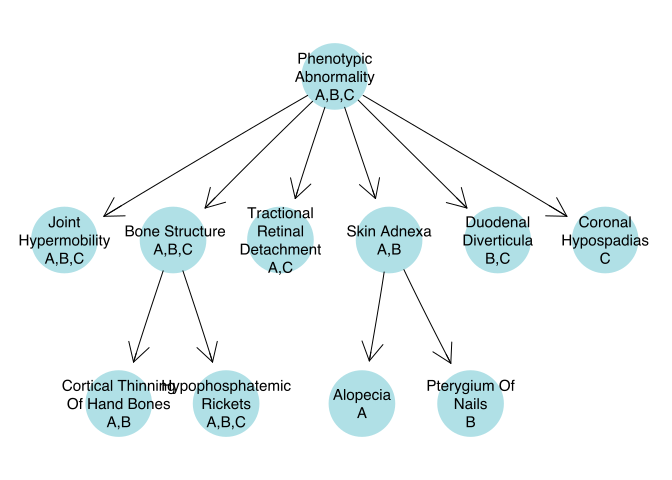To further decorate the plot, we could use the frequency of each term in hpo_phenotypes them to colour them.

onto_plot(
hpo,
frequencies=get_term_frequencies(hpo, hpo_phenotypes),
term_sets=hpo_phenotypes,
label=label_by_term_set,
fillcolor=colour_by_frequency)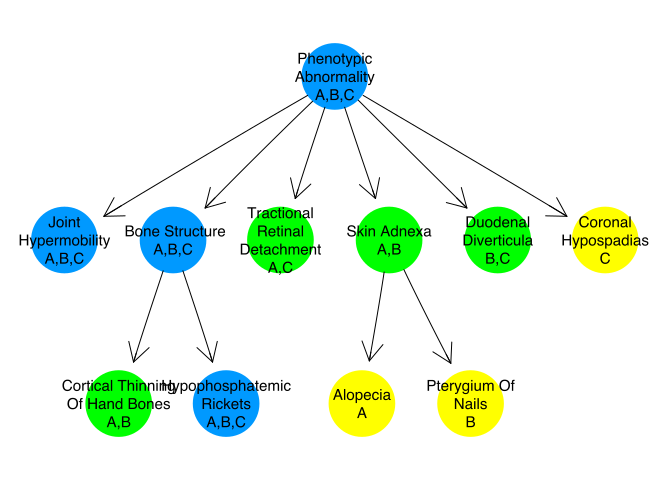Edge attributes

Edge attributes recognised by Rgraphviz can also be supplied by the edge_attributes argument.

onto_plot(
hpo,
frequencies=get_term_frequencies(hpo, hpo_phenotypes),
term_sets=hpo_phenotypes,
label=label_by_term_set,
edge_attributes=list(color="red", lty="dashed"))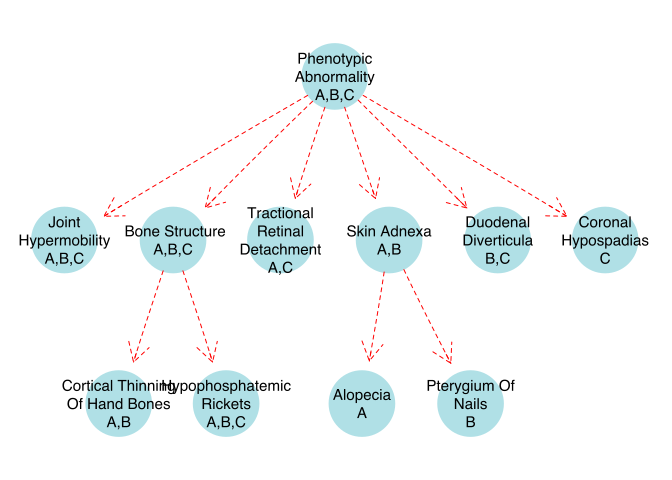Plots for publications

To get the highest quality plots and fine-grained control over the node and edge attributes, the best option is to create the ontological_plot with onto_plot and write it to a file in dot format using the write_dot function. The file can then be opened using a layout program, for example the graphviz program (http://www.graphviz.org/), to visualise/export as an image. Individual node and edge attributes can then be modified by editing the file.